Mobile QR Code1. (Department of Electronic and Electrical Engineering, POSTECH (Pohang University of Science and Technology), Pohang 37673, Korea )

DRAM controller, ASIC, low power, termination, signal integrity

## I. INTRODUCTION

Recently, deep learning has been widely adopted in mobile electronic devices for applications such as image or speech recognition. The mobile devices accept the input data, send them to cloud computers via a wireless communication network for deep-learning processing, receive the processed data from the cloud computers, and display images or generate sounds at the mobile devices for the received data [1,2]. This method has a latency problem associated with wireless communication to and from cloud computers. Also, data privacy is an issue in this method, because all the user data are sent to a cloud computer for deep learning processing. To avoid latency and privacy issues, on-device deep-learning mobile devices are actively studied at the research level [3,4]. The on-device deep learning requires a large-size memory with high bandwidth as well as a fast processing element with high processing power; a commercial dynamic random access memory (DRAM) chip is the most suitable memory for this application. Hence, the on-device deep-learning hardware mostly consists of an application-specific integrated circuit (ASIC) chip and commercial DRAM chips; the ASIC chip includes a processing element and a DRAM controller circuit [5,6].

There are three issues to be considered in implementing the DRAM controller in an ASIC chip for on-device deep learning.

The first issue is that the DRAM controller requires many pin counts to communicate with commercial DRAM chips. For example, the DRAM controller for a 16 DQ 8 Gb DDR3 DRAM chip needs 48 pins for signaling (16 DQ, 4 DQS, 16 address, 3 bank address, 2~CK, 7 commands (RASN, CASN, WEN, CSN, CKE, ODT, RESETN)) .

The second issue is that the DRAM controller occupies a significant portion of the ASIC chip area. The large pin count increases chip area because each I/O signal requires a large buffer circuit.

The third issue, the most important one, is that the DRAM and the DRAM controller consume a large power; the DRAM and the DRAM controller consume 41 ~ 59% in  and 50 ~ 70% in  of the total power in on-device deep learning applications. Because the DRAM interface uses a high data rate, termination is required at the transceiver to reduce reflection. The DDR3 SDRAM chip uses on-die termination resistors of 60 ${\Omega}$ for write and 34 ${\Omega}$ for read operations in default, respectively, by following the Joint Electron Device Engineering Council (JEDEC) standard . Due to the termination, the transceiver consumes large static power; the transceiver blocks of the DRAM controller and the DRAM chip consume 110 mW and 67 mW, respectively . Because the static power of the transceiver is inversely proportional to the termination resistance, we can reduce the static power by increasing the on-die termination resistance . Increasing the termination resistance degrades the signal integrity due to reflections at chip pins because the characteristic impedance of transmission lines on printed circuit board (PCB) is constant at 50 ${\Omega}$ mostly. To avoid signal integrity degradation, short-reach interconnects are used for the transmission lines on PCB.

Section II describes issues for power consumption of the DDR3 DRAM interface system. Section III presents the measured results of the DRAM controller. Section IV presents the application of this work to the long-reach point-to-point interface and the multi-drop DRAM interface. Section V concludes this work.

## II. POWER CONSUMPTION OF DDR3 DRAM INTERFACE SYSTEM

Among the three issues considered in the Introduction to implementing the DRAM controller in an ASIC chip, this work focused on reducing power consumption. To implement a low-power DRAM controller, a double data rate 3 (DDR3) DRAM controller was chosen in this work. As shown in Fig. 1, the DDR3 DRAM controller consists of a DRAM controller core and a test module. The DRAM controller core consists of a LINK and three branches; an ADDR/CMD branch, a DQ/DQS write branch, and a DQ/DQS read branch. Each branch consists of 4:1 serializers or deserializers and I/O circuits. The LINK performs the master control functions of the DRAM controller, such as initialization, data read/write, and refresh operations. To achieve the higher bandwidth, the LINK which consists of complex logic circuits runs at a 4 times slower clock than the data rate of DQs. In order to compensate the frequency difference between the LINK and the I/O circuits, 4:1 serializers and deserializers are added between the LINK and the I/O circuits.

Among the elements of the DRAM controller core (Fig. 1), the I/O circuits for DQ/DQS are the most power-hungry, as shown in Table 1.

The power consumption of I/O circuits is dominated by the current required to drive the small termination resistance with a large voltage swing, which should be large enough for the receiver circuit to recover the digital data within a short period of data rate (one data UI). To avoid reflections of long transmission lines on PCB, the termination resistance is mostly set to the characteristic impedance of the transmission line, which is around 50 ${\Omega}$.

However, for short-reach interconnects, increasing the termination resistance to a value larger than the characteristic impedance of the transmission line does not bring out serious signal integrity problems. Although increasing the termination resistance reduces the I/O power, for some combinations of termination resistance at the transmitter (TX) and receiver (RX), the signal swing at RX falls below the minimum RX voltage swing required for the error-free recovery of digital data. The minimum RX voltage swing is defined by the JEDEC standard .

The I/O circuits of the DRAM controller in this paper are designed such that the termination resistance can be changed by the external register control. The TX driver is implemented by eight parallel tri-state buffer cells (Fig. 2(a)). The resistance of each NMOS and PMOS of the buffer cell is set to 480 ${\Omega}$, so the TX termination resistance (R$_{\mathrm{TX}}$) ranges from 60 ${\Omega}$ to 480 ${\Omega}$ by the 7-bit thermometer control with the cell 1 turned on in the write mode. The RX buffer is implemented by a conventional Gunning Transceiver Logic (GTL) circuit and seven parallel tri-state buffer cells (Fig. 2(b)); the buffer cells work as the RX termination resistance (R$_{\mathrm{RX}}$). The resistance of each NMOS and PMOS of the buffer cell is set to 480 ${\Omega}$ so that R$_{\mathrm{RX}}$ ranges from 34 ${\Omega}$ to infinity by the 7-bit thermometer control.

For the commercial DRAM chip, R$_{\mathrm{TX}}$ and R$_{\mathrm{RX}}$ can be changed by the mode register set (MRS); R$_{\mathrm{TX}}$ is set to 34 or 40 ${\Omega}$ and R$_{\mathrm{RX}}$ ranges from 20 to 120 ${\Omega}$. However, R$_{\mathrm{TX}}$ and R$_{\mathrm{RX}}$ of the DRAM chip are set to their default values, 34 ${\Omega}$ and 60 ${\Omega}$, respectively, to focus on the DRAM controller in this work.

The DDR3 DRAM interface adopts the center tap terminated (CTT) logic, as shown in Fig. 3(a), by following the JEDEC standard; at TX, the transmission line is connected to VDDQ when D$_{\mathrm{in}}$(t) is ’+1’ and to ground when D$_{\mathrm{in}}$(t) is ’-1’. The total power is a multiplication of I$_{\mathrm{TX}}$+I$_{\mathrm{RX}}$ and VDDQ (Eq. (1)); I$_{\mathrm{TX}}$ is the supply current of the TX driver and I$_{\mathrm{RX}}$ is the supply current of the RX buffer. In the AC equivalent circuit of the DDR3 DRAM interface in Fig. 3(b), the transmission line is a uniformly distributed LC line, where the (V, I) wave propagates along the line.

The TX supply current I$_{\mathrm{TX}}$(t) is determined by the input data D$_{\mathrm{in}}$(t) and the incident and reflected current waves at TX, I$_{\mathrm{I,TX}}$ and I$_{\mathrm{R,TX}}$, as in Eq. (2); D$_{\mathrm{in}}$(t) is either ‘+1’ or ‘-1’ and I$_{\mathrm{TX}}$(t) is 0 when D$_{\mathrm{in}}$(t) is ‘-1’. The RX supply current I$_{\mathrm{RX}}$(t) is derived as Eq. (3) by applying the Kirchhoff voltage and current laws at the RX node; I$_{\mathrm{I,RX}}$ and I$_{\mathrm{R,RX}}$ is the incident and reflected current waves at RX, respectively. ${\Gamma}$$_{\mathrm{T}} and {\Gamma}$$_{\mathrm{R}}$ are the reflection coefficients at TX and RX, respectively (Eqs. (4) and (5)).

##### (1)
$\begin{array} \text{Power}&=\text{VDDQ}\cdot \left(\mathrm{I}_{\mathrm{TX}}+\mathrm{I}_{\mathrm{RX}}\right) \\ \end{array}$
##### (2)
$\begin{array} \mathrm{I}_{\mathrm{TX}}\left(\mathrm{t}\right)&=\frac{\mathrm{D}_{\mathrm{in}}\left(\mathrm{t}\right)+1}{2}\cdot \left[\mathrm{I}_{\mathrm{I}.\mathrm{TX}}\left(\mathrm{t}\right)-\mathrm{I}_{\mathrm{R}.\mathrm{TX}}\left(\mathrm{t}\right)\cdot \left(1-\Gamma _{\mathrm{T}}\right)\right] \\ \end{array}$
##### (3)
$\begin{array} \mathrm{I}_{\mathrm{RX}}\left(\mathrm{t}\right)&=\frac{\text{VDDQ}}{4\mathrm{R}_{\mathrm{RX}}}-\frac{1}{2}\left[\mathrm{I}_{\mathrm{I},\mathrm{RX}}\left(\mathrm{t}\right)-\mathrm{I}_{\mathrm{R},\mathrm{RX}}\left(\mathrm{t}\right)\right]\cdot \left(1-\Gamma _{\mathrm{R}}\right) \\ \end{array}$
##### (4)
$\begin{array} \mathrm{\Gamma}_{\mathrm{T}}&=\frac{\mathrm{R}_{\mathrm{TX}}-\mathrm{Z}_{\mathrm{o}}}{\mathrm{R}_{\mathrm{TX}}+\mathrm{Z}_{\mathrm{o}}} \\ \end{array}$
##### (5)
$\begin{array} \mathrm{\Gamma}_{\mathrm{R}}&=\frac{\mathrm{R}_{\mathrm{RX}}-\mathrm{Z}_{\mathrm{o}}}{\mathrm{R}_{\mathrm{RX}}+\mathrm{Z}_{\mathrm{o}}} \end{array}$

I$_{\mathrm{I,TX}}$ is determined by the input data D$_{\mathrm{in}}$(t) as in Eq. (6); the transmission line can be considered as a grounded resistance of Z$_{\mathrm{o}}$ for a fast transition signal D$_{\mathrm{in}}$(t).

##### (6)
$\mathrm{I}_{\mathrm{I}.\mathrm{TX}}\left(\mathrm{t}\right)=\frac{\text{VDDQ}}{2\left(\mathrm{R}_{\mathrm{TX}}+\mathrm{Z}_{\mathrm{o}}\right)}\cdot \mathrm{D}_{\mathrm{in}}\left(\mathrm{t}\right)$

I$_{\mathrm{R,TX}}$ is the summation of multiple reflections as in Eq. (7); t$_{\mathrm{f}}$ is the time of flight of the transmission line.

##### (7)
$\mathrm{I}_{\mathrm{R}.\mathrm{TX}}\left(\mathrm{t}\right)=\sum _{\mathrm{m}=1}^{\infty }\left\{\mathrm{I}_{\mathrm{I}.\mathrm{TX}}\left(\mathrm{t}-2\mathrm{mt}_{\mathrm{f}}\right)\cdot \left(\Gamma _{\mathrm{R}}\Gamma _{\mathrm{T}}\right)^{\mathrm{m}-1}\Gamma _{\mathrm{R}}\right\}$

By substituting Eq. (6) and Eq. (7) into Eq. (2), I$_{\mathrm{TX}}$ equation is derived as in Eq. (8).

##### (8)
$\begin{array}{l} \mathrm{I}_{\mathrm{TX}}\left(\mathrm{t}\right)=\frac{\text{VDDQ}}{4\left(\mathrm{R}_{\mathrm{TX}}+\mathrm{Z}_{\mathrm{o}}\right)}\cdot \left[\mathrm{D}_{\mathrm{in}}\left(\mathrm{t}\right)^{2}+\mathrm{D}_{\mathrm{in}}\left(\mathrm{t}\right)-\sum _{\mathrm{m}=1}^{\infty }\left\{\mathrm{D}_{\mathrm{in}}\left(\mathrm{t}\right)\cdot \right.\right.\\ \left.\left.\left(\mathrm{D}_{\mathrm{in}}\left(\mathrm{t}-2\mathrm{mt}_{\mathrm{f}}\right)+\mathrm{D}_{\mathrm{in}}\left(\mathrm{t}-2\mathrm{mt}_{\mathrm{f}}\right)\right)\cdot \left(\Gamma _{\mathrm{R}}\Gamma _{\mathrm{T}}\right)^{\mathrm{m}-1}\Gamma _{\mathrm{R}}\left(1-\Gamma _{\mathrm{T}}\right)\right\}\right] \end{array}$

I$_{\mathrm{I,RX}}$ in Eq. (3) is the first time incident waveform at RX, that is I$_{\mathrm{I.TX}}$ delayed by t$_{\mathrm{f}}$, as in Eq. (9). All the reflected waveforms are included in I$_{\mathrm{R.RX}}$, as in Eq. (10).

##### (9)
$\begin{array} \mathrm{I}_{\mathrm{I}.\mathrm{RX}}\left(\mathrm{t}\right)&=\mathrm{I}_{\mathrm{I}.\mathrm{TX}}\left(\mathrm{t}-\mathrm{t}_{\mathrm{f}}\right) \\ \end{array}$
##### (10)
$\begin{array} \mathrm{I}_{\mathrm{R}.\mathrm{RX}}\left(\mathrm{t}\right)&=-\sum _{\mathrm{m}=1}^{\infty }\left\{\mathrm{I}_{\mathrm{I},\mathrm{TX}}\left(\mathrm{t}-\left(2\mathrm{m}+1\right)\mathrm{t}_{\mathrm{f}}\right)\cdot \left(\Gamma _{\mathrm{R}}\Gamma _{\mathrm{T}}\right)^{\mathrm{m}}\right\} \end{array}$

By substituting Eq. (9) and Eq. (10) into Eq. (3), the I$_{\mathrm{RX}}$ equation is derived as in Eq. (11).

##### (11)
$\begin{array}{l} \mathrm{I}_{\mathrm{RX}}\left(\mathrm{t}\right)=\frac{\text{VDDQ}}{4\mathrm{R}_{\mathrm{RX}}}-\frac{\text{VDDQ}}{4\left(\mathrm{R}_{\mathrm{TX}}+\mathrm{Z}_{\mathrm{o}}\right)}\cdot \left[\mathrm{D}_{\mathrm{in}}\left(\mathrm{t}-\mathrm{t}_{\mathrm{f}}\right)+\right.\\ \sum _{\mathrm{m}=1}^{\infty }\left\{\mathrm{D}_{\mathrm{in}}\left(\mathrm{t}-\left(2\mathrm{m}+1\right)\mathrm{t}_{\mathrm{f}}\right)\cdot \right.\left.\left.\left(\Gamma _{\mathrm{R}}\Gamma _{\mathrm{T}}\right)^{\mathrm{m}}\right\}\right]\cdot \left(1-\Gamma _{\mathrm{R}}\right) \end{array}$

To get the average supply power Eq. (1), the long-term time average values of I$_{\mathrm{TX}}$ and I$_{\mathrm{RX}}$ are derived from Eqs. (8) and (11), respectively. Two cases of D$_{\mathrm{in}}$(t) are considered in this derivation; one is a random binary sequence of ‘+1’ and ‘-1’ such as a pseudo-random binary sequence (PRBS) data and the other is a clock signal which repeats the binary sequence of ‘+1’ followed by ‘-1’ indefinitely in time.

In both cases, the long-term time average values of D$_{\mathrm{in}}$(t), D$_{\mathrm{in}}$(t-2mt$_{\mathrm{f}}$) and D$_{\mathrm{in}}$(t)$^{2}$ at Eq. (8) are 0, 0 and 1, respectively. D$_{\mathrm{in}}$(t-2mt$_{\mathrm{f}}$) is D$_{\mathrm{in}}$(t) delayed by 2mt$_{\mathrm{f}}$; it arrives at TX after the m-th round trip along the transmission line. The long-term time average of D$_{\mathrm{in}}$(t)${\cdot}$D$_{\mathrm{in}}$(t-2mt$_{\mathrm{f}}$) has different values in the two cases. For the case of the random sequence D$_{\mathrm{in}}$(t), when the m-th reflection arrives within one data period (2mt$_{\mathrm{f}}${\textless}t$_{\mathrm{ui}}$), D$_{\mathrm{in}}$(t) and D$_{\mathrm{in}}$(t-2mt$_{\mathrm{f}}$) are uncorrelated during the initial 2mt$_{\mathrm{f}}$ time interval of one t$_{\mathrm{ui}}$, and they have the same values of either ‘+1’ or ‘-1’ during the remaining t$_{\mathrm{ui}}$-2mt$_{\mathrm{f}}$ time interval, as shown in Fig. 4(a), where D$_{\mathrm{in}}$(t) is assumed to be ‘+1’ during the one t$_{\mathrm{ui}}$ time interval considered. Thus, for 2mt$_{\mathrm{f}}${\textless}t$_{\mathrm{ui}}$, the long-term time average of D$_{\mathrm{in}}$(t)${\cdot}$D$_{\mathrm{in}}$(t-2mt$_{\mathrm{f}}$) is (t$_{\mathrm{ui}}$-2mt$_{\mathrm{f}}$)/t$_{\mathrm{ui}}$ as in the first part of Eq. (12). When the m-th reflection arrives after one data period (2mt_{\mathrm{f}}{\geq}t_{\mathrm{ui}}), D_{\mathrm{in}}(t) and D_{\mathrm{in}}(t-2mt_{\mathrm{f}}) are uncorrelated during the time interval of one t_{\mathrm{ui}}, as shown in Fig. 4(b), the long-term time average of D_{\mathrm{in}}(t){\cdot}D_{\mathrm{in}}(t-2mt_{\mathrm{f}}) is 0 as in the second part of Eq. (12). ##### (12) \mathrm{AVG}\left(\mathrm{D}_{\mathrm{in}}\left(\mathrm{t}\right)\cdot \mathrm{D}_{\mathrm{in}}\left(\mathrm{t}-2\mathrm{mt}_{\mathrm{f}}\right)\right)=\left\{\begin{array}{ll} \frac{\mathrm{t}_{\mathrm{ui}}-2\mathrm{mt}_{\mathrm{f}}}{\mathrm{t}_{\mathrm{ui}}}, & 2\mathrm{mt}_{\mathrm{f}}<\mathrm{t}_{\mathrm{ui}}\\ 0, & 2\mathrm{mt}_{\mathrm{f}}\geq \mathrm{t}_{\mathrm{ui}} \end{array}\right. By substituting Eq. (12) into Eq. (8), the long-term time average of I_{\mathrm{TX}} for the random binary sequence D_{\mathrm{in}}(t) is given by Eq. (13). ##### (13) \begin{align} \begin{array}{l} \mathrm{I}_{\mathrm{TX}.\mathrm{AVG}.\text{RANDOM}}=\\ \frac{\text{VDDQ}}{4\left(\mathrm{R}_{\mathrm{TX}}+\mathrm{Z}_{\mathrm{o}}\right)}\left[1-\sum _{\mathrm{m}=1}^{\mathrm{M}}\left\{\left(1-\frac{2\mathrm{mt}_{\mathrm{f}}}{\mathrm{t}_{\mathrm{ui}}}\right)\left(\Gamma _{\mathrm{R}}\Gamma _{\mathrm{T}}\right)^{\mathrm{m}-1}\Gamma _{\mathrm{R}}\left(1-\Gamma _{\mathrm{T}}\right)\right\}\right] \end{array} \end{align} ##### (14) \begin{align} \begin{array}{l} \mathrm{M}&=\text{floor}\left(\frac{\mathrm{t}_{\mathrm{ui}}}{2\mathrm{t}_{\mathrm{f}}}\right) \end{array} \end{align} The floor function of Eq. (14) returns the largest integer which is equal to or smaller than the input argument. For the case of the clock signal D_{\mathrm{in}}(t), which is ‘+1’ during the time interval of one t_{\mathrm{ui}} and ‘-1’ during the following t_{\mathrm{ui}}, the long-term time average of D_{\mathrm{in}}(t){\cdot}D_{\mathrm{in}}(t-2mt_{\mathrm{f}}) can be calculated in two cases; in the first case (Fig. 5(a)), the rising edge of the reflected clock signal D_{\mathrm{in}}(t-2mt_{\mathrm{f}}) arrives at TX while D_{\mathrm{in}}(t) is ‘+1’, and in the second case (Fig. 5(b)), the rising edge of D_{\mathrm{in}}(t-2mt_{\mathrm{f}}) arrives at TX while D_{\mathrm{in}}(t) is ‘-1’. A normalized variable x, as defined in Eq. (15), ranges from 0 to 0.5 for the first case and it ranges from 0.5 to 1.0 for the second case. The time-average of D_{\mathrm{in}}(t){\cdot}D_{\mathrm{in}}(t-2mt_{\mathrm{f}}) can be derived as f(x) shown in Eq. (16) for the case of the clock signal D_{\mathrm{in}}(t). ##### (15) \begin{align} \mathrm{x}&=\frac{\mathrm{mt}_{\mathrm{f}}}{\mathrm{t}_{\mathrm{ui}}}-\text{floor}\left(\frac{\mathrm{mt}_{\mathrm{f}}}{\mathrm{t}_{\mathrm{ui}}}\right) \\ \end{align} ##### (16) \begin{align} \begin{array}{l} \mathrm{AVG}\left(\mathrm{D}_{\mathrm{in}}\left(\mathrm{t}\right)\cdot \mathrm{D}_{\mathrm{in}}\left(\mathrm{t}-2\mathrm{mt}_{\mathrm{f}}\right)\right)=\mathrm{f}\left(\mathrm{x}\right)=\\ \left\{\begin{array}{ll} 1-4\mathrm{x}, & 0\leq \mathrm{x}<0.5\\ 4\mathrm{x}-3, & 0.5\leq \mathrm{x}<1 \end{array}\right. \end{array} \end{align} Substitution of Eq. (16) into Eq. (8) yields the long-term time average of I_{\mathrm{TX}} for the clock signal D_{\mathrm{in}}(t) as Eq. (17). ##### (17) \begin{array}{l} \mathrm{I}_{\mathrm{TX}.\mathrm{AVG}.\text{CLOCK}}=\\ \frac{\text{VDDQ}}{4\left(\mathrm{R}_{\mathrm{TX}}+\mathrm{Z}_{\mathrm{o}}\right)}\left[1-\sum _{\mathrm{m}=1}^{\infty }\left\{\mathrm{f}\left(\mathrm{x}\right)\cdot \left(\Gamma _{\mathrm{R}}\Gamma _{\mathrm{T}}\right)^{\mathrm{m}-1}\Gamma _{\mathrm{R}}\left(1-\Gamma _{\mathrm{T}}\right)\right\}\right] \end{array} The long-term time average of I_{\mathrm{RX}} is derived as Eq. (18) independently of D_{\mathrm{in}}(t), because the long-term time average values of D_{\mathrm{in}}(t-t_{\mathrm{f}}) and D_{\mathrm{in}}(t-(2m+1)t_{\mathrm{f}}) in Eq. (11) are all 0 for both the random binary sequence and the clock signal D_{\mathrm{in}}(t). ##### (18) \mathrm{I}_{\mathrm{RX}.\mathrm{AVG}}\left(\mathrm{t}\right)=\frac{\text{VDDQ}}{4\mathrm{R}_{\mathrm{RX}}} Fig. 6 shows the long-term time average of the supply current I_{\mathrm{TX}}+I_{\mathrm{RX}} versus the length of the transmission line; a lossless transmission line is assumed. For a given length of transmission line, the supply current is reduced by increasing either R_{\mathrm{TX}} or R_{\mathrm{RX}}. The calculation using Eqs. (13), (17) and (18) yields an absolute error of less than 0.8% from the SPICE simulation; t_{\mathrm{ui}} is 0.5 ns and t_{\mathrm{f}} is calculated using the propagation velocity along the transmission line of 1.711*10^{10} cm/s assuming a microstrip transmission line on FR-4 PCB. If t_{\mathrm{f}}=0, that is, no transmission line is used, the long-term time average I_{\mathrm{TX}} equations Eqs. (13) and (17) should be reduced to the average DC current equation 0.25VDDQ/(R_{\mathrm{TX}}+R_{\mathrm{RX}}). Because t_{\mathrm{f}}=0 gives (t_{\mathrm{ui}}-2mt_{\mathrm{f}})/t_{\mathrm{ui}}=1, M=infinity, x=0, f(x)=1, Eqs. (13) and (17) result in the same equation Eq. (19), which agrees with the DC current equation. This verifies the validity of Eqs. (13) and (17) for the DC case. ##### (19) \begin{array}{l} \mathrm{I}_{\mathrm{TX}.\mathrm{AVG}}\left(\mathrm{t}_{\mathrm{f}}=0\right)=\\ \frac{\text{VDDQ}}{4\left(\mathrm{R}_{\mathrm{TX}}+\mathrm{Z}_{\mathrm{o}}\right)}\left[1-\sum _{\mathrm{m}=1}^{\lesseqgtr }\left\{\left(\Gamma _{\mathrm{R}}\Gamma _{\mathrm{T}}\right)^{\mathrm{m}-1}\Gamma _{\mathrm{R}}\left(1-\Gamma _{\mathrm{T}}\right)\right\}\right]=\frac{\text{VDDQ}}{4\left(\mathrm{R}_{\mathrm{TX}}+\mathrm{R}_{\mathrm{RX}}\right)} \end{array} Eq. (19) corresponds to the minimum value of Eqs. (13) and (17) for all t_{\mathrm{f}} if {\Gamma}_{\mathrm{T}}$${\geq}0 and {\Gamma}$$_{\mathrm{R}}$${\geq}0. In Fig. 6, we can observe the periodic change of I_{\mathrm{TX}}+I_{\mathrm{RX}} w.r.t. the length of the transmission line for the clock signal D_{\mathrm{in}}(t); I_{\mathrm{TX}}+I_{\mathrm{RX}} is minimized at 2t_{\mathrm{f}}=2nt_{\mathrm{ui}} and maximized at 2t_{\mathrm{f}}=(2n+1)t_{\mathrm{ui}}; n is an integer. This is due to the time synchronization of the incident and reflected current waves at TX; they are synchronized in the same phase at 2t_{\mathrm{f}}=2nt_{\mathrm{ui}} (Fig. 7(a)) and in the alternately opposite phase at 2t_{\mathrm{f}}=(2n+1)t_{\mathrm{ui}} (Fig. 7(b)). Both R_{\mathrm{TX}} and R_{\mathrm{RX}} are assumed to be larger than Z_{\mathrm{o}}. Because I_{\mathrm{TX}} is the difference between the incident and reflected current waves as in Eq. (3), the same phase synchronization gives the minimum I_{\mathrm{TX}} as in Eq. (19) and the alternately opposite phase synchronization gives the maximum I_{\mathrm{TX}} as in Eq. (20). ##### (20) \begin{array}{l} \mathrm{I}_{\mathrm{TX}.\mathrm{AVG}}\left(\mathrm{t}_{\mathrm{f}}=\left(\mathrm{n}+0.5\right)\mathrm{t}_{\mathrm{ui}}\right)=\\ \frac{\text{VDDQ}}{4\left(\mathrm{R}_{\mathrm{TX}}+\mathrm{Z}_{\mathrm{o}}\right)}\left[1+\sum _{\mathrm{m}=1}^{\infty }\left\{\left(-\Gamma _{\mathrm{R}}\Gamma _{\mathrm{T}}\right)^{\mathrm{m}-1}\Gamma _{\mathrm{R}}\left(1-\Gamma _{\mathrm{T}}\right)\right\}\right]=\frac{\text{VDDQ}}{4\left(\mathrm{R}_{\mathrm{TX}}+\frac{{\mathrm{Z}_{\mathrm{o}}}^{2}}{\mathrm{R}_{\mathrm{RX}}}\right)} \end{array} Although increasing R_{\mathrm{TX}} or R_{\mathrm{RX}} reduces the power consumption of the DRAM interface (Fig. 3) as dictated by Eqs. (13), (17) and (18), some combinations of R_{\mathrm{TX}} or R_{\mathrm{RX}} cannot meet the minimum RX voltage swing which is required to retrieve the correct digital data at RX within t_{\mathrm{ui}}; it is defined to be V_{\mathrm{ref}} {\pm} 0.1VDDQ for DDR3 DRAM chips by the JEDEC standard with VDDQ=1.5 V and V_{\mathrm{ref}}=0.75 V. The RX voltage swing is determined by the transmission line effects such as reflections as well as the termination resistors (R_{\mathrm{TX}}, R_{\mathrm{RX}}). With R_{\mathrm{TX}}=34 {\Omega} and R_{\mathrm{RX}}=60 {\Omega} for the DDR3 DRAM chip, the R_{\mathrm{TX}} of the DRAM controller cannot exceed 240 {\Omega} and R_{\mathrm{RX}} can be increased indefinitely to maintain the minimum DC RX voltage swing, as can be seen in Table 2. To find the reduction of the RX voltage swing by the reflections due to the unmatched R_{\mathrm{TX}} and R_{\mathrm{RX}}, chip package and vias, SPICE simulation is performed for the circuit model of the DDR3 DRAM interface (Fig. 8). The circuit model includes the TQFP176 package  for the DRAM controller, the pi via model , the IBIS model of the commercial DDR3 DRAM chip , the RLGC parameters of a microstrip line extracted from the measured S-parameters. Fig. 9 shows the eye diagram of the simulated RX voltage with D_{\mathrm{in}}(t)=2.133 Gbps PRBS-15 and the length of the transmission line 5 cm. The controller R_{\mathrm{TX}}=240 {\Omega} cannot meet the RX eye mask requirement of 300 mV and 280 ps (Fig. 9(a)), while the controller R_{\mathrm{TX}}=160 {\Omega} satisfies the requirement (Fig. 9(b)). The controller R_{\mathrm{RX}}=infinity satisfies the requirement (Fig. 9(c)). From the RX voltage swing from SPICE simulation w.r.t. the length of the transmission line for different values of controller R_{\mathrm{TX}} and R_{\mathrm{RX}} shown in Fig. 10, we can increase the length of the transmission line up to 6~cm at write mode with the controller R_{\mathrm{TX}}=160 {\Omega} with DRAM R_{\mathrm{RX}}=60 {\Omega}. Also, we can use a long transmission line and a large controller R_{\mathrm{RX}} at read mode with DRAM R_{\mathrm{TX}}=34 {\Omega}. ##### Table 1. Power consumption of the DRAM interface including DRAM and controller (HSPICE simulation for I/O circuits and IC compiler reports for logic circuits, 60 {\Omega} termination for write and 34 {\Omega} termination for read)  DQ/DQS circuits (DRAM) 177 mW DQ/DQS circuits (controller) 239 mW ADDR/CMD drivers & logic (DRAM) 99 mW ADDR/CMD drivers & logic (controller) 99 mW Total power 614 mW ##### Table 2. DC RX voltage level and swing  RX voltage level, Din='-1' RX voltage level, Din='+1' RX voltage swing \frac{\text{VDDQ}\cdot \mathrm{R}_{\mathrm{TX}}}{2\left(\mathrm{R}_{\mathrm{TX}}+\mathrm{R}_{\mathrm{RX}}\right)} \frac{\text{VDDQ}\cdot \left(\mathrm{R}_{\mathrm{TX}}+2\mathrm{R}_{\mathrm{RX}}\right)}{2\left(\mathrm{R}_{\mathrm{TX}}+\mathrm{R}_{\mathrm{RX}}\right)} \frac{\text{VDDQ}\cdot \mathrm{R}_{\mathrm{RX}}}{\mathrm{R}_{\mathrm{TX}}+\mathrm{R}_{\mathrm{RX}}} ##### Fig. 1. Block diagram of DDR3 DRAM controller ASIC connected to an external DDR3 DRAM chip.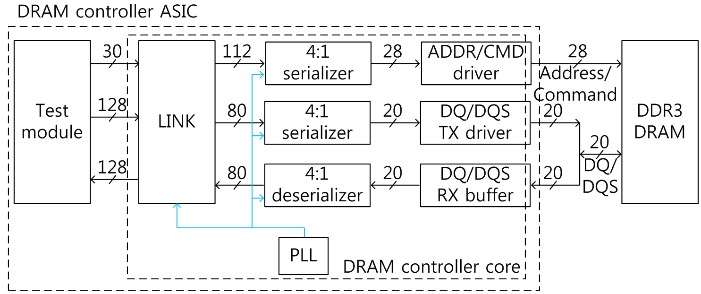##### Fig. 2. I/O circuits of DDR3 DRAM controller: (a) TX driver; (b) RX buffer.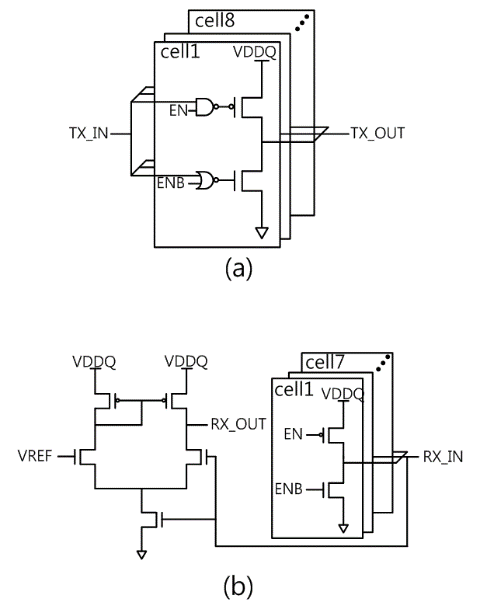##### Fig. 3. I/O circuits of DDR3 DRAM controller: (a) TX driver; (b) RX buffer.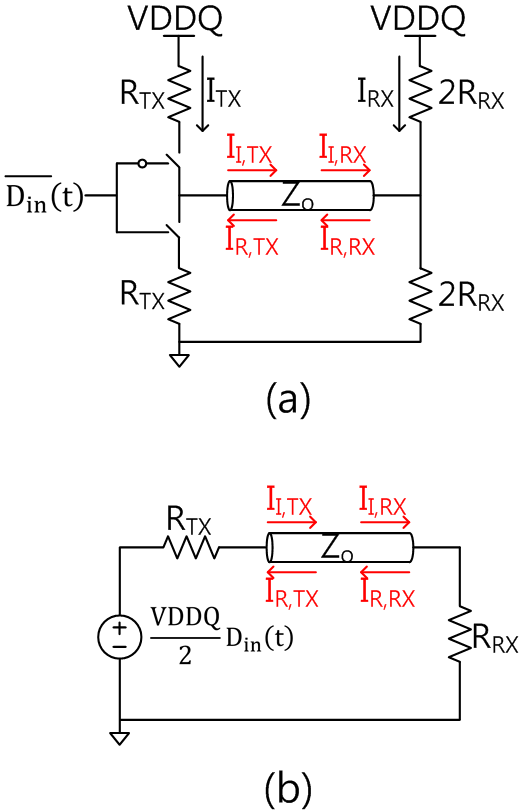##### Fig. 4. Waveforms for random binary sequence D_{\mathrm{in}}(t): (a) 2mt_{\mathrm{f}}{\textless}t_{\mathrm{ui}}; (b) 2mt_{\mathrm{f}}$${\geq}t$_{\mathrm{ui}}$.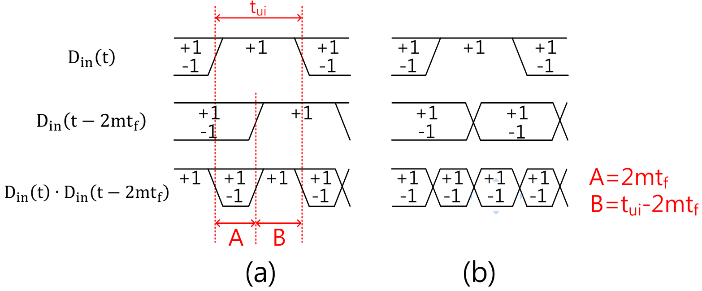##### Fig. 5. Waveforms for clock signal D$_{\mathrm{in}}$(t): (a) x{\textless}0.5; (b) x${\geq}$0.5; x is the normalized t$_{\mathrm{f}}$ as in Eq. (15).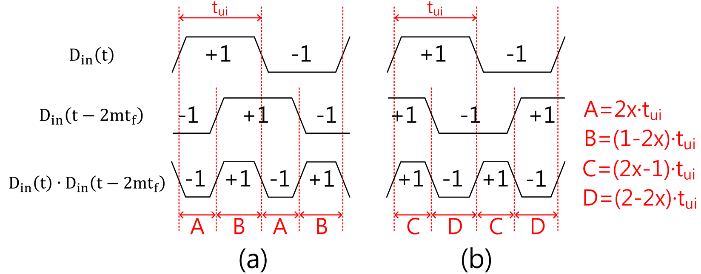##### Fig. 6. Long-term time average of I$_{\mathrm{TX}}$+I$_{\mathrm{RX}}$ versus the length of transmission line for calculation (solid line) and SPICE simulation (symbol): (a) PRBS-7 D$_{\mathrm{in}}$(t); (b) clock D$_{\mathrm{in}}$(t).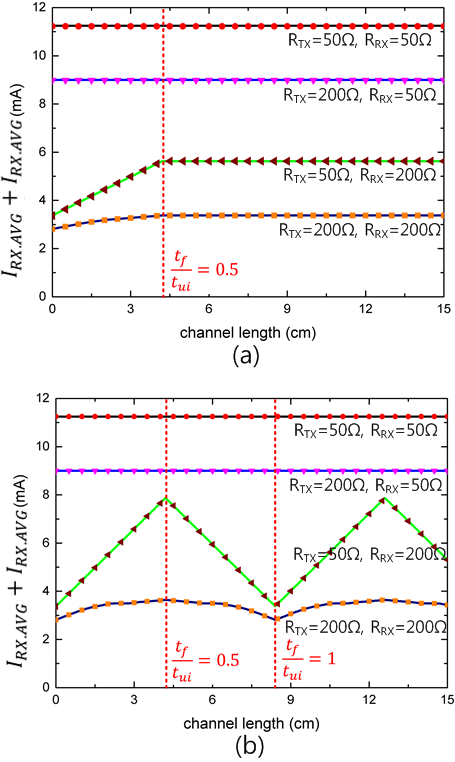##### Fig. 7. Waveforms of the incident current wave, reflected current waves and I$_{\mathrm{TX}}$ for clock signal D$_{\mathrm{in}}$(t) while D$_{\mathrm{in}}$(t)=‘+1’: (a) 2t$_{\mathrm{f}}$=2nt$_{\mathrm{ui}}$; (b) 2t$_{\mathrm{f}}$=(2n+1)t$_{\mathrm{ui}}$.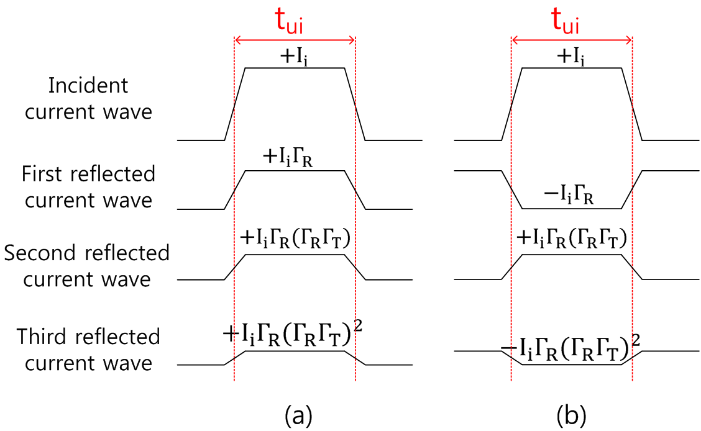##### Fig. 8. Circuit model of DDR3 DRAM interface with PCB routing and chip package models: (a) write mode; (b) read mode.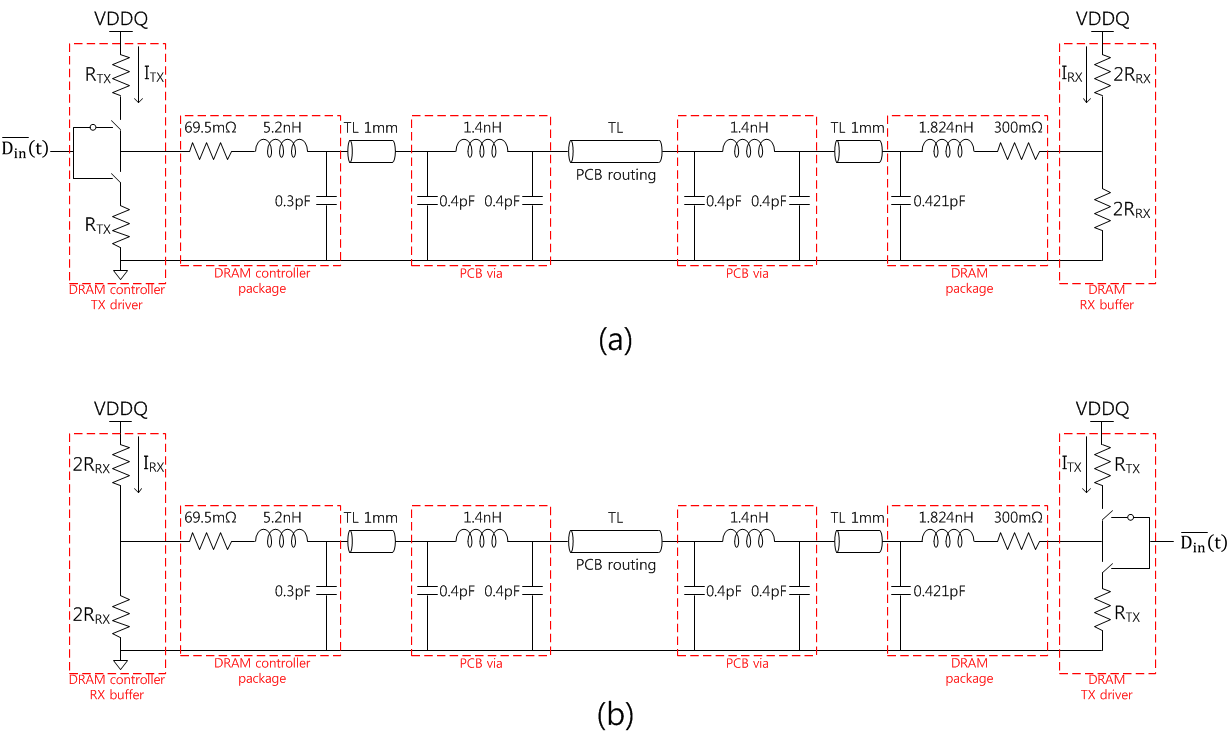##### Fig. 9. Eye diagrams of DDR3 DRAM interface for 2.133 Gbps PRBS-15 input: (a) write mode, controller R$_{\mathrm{TX}}$=240 ${\Omega}$, DRAM R$_{\mathrm{RX}}$=60 ${\Omega}$; (b) write mode, controller R$_{\mathrm{TX}}$=160 ${\Omega}$, DRAM R$_{\mathrm{RX}}$=60 ${\Omega}$; (c) read mode, controller R$_{\mathrm{RX}}$=infinity(open), DRAM R$_{\mathrm{TX}}$=34 ${\Omega}$.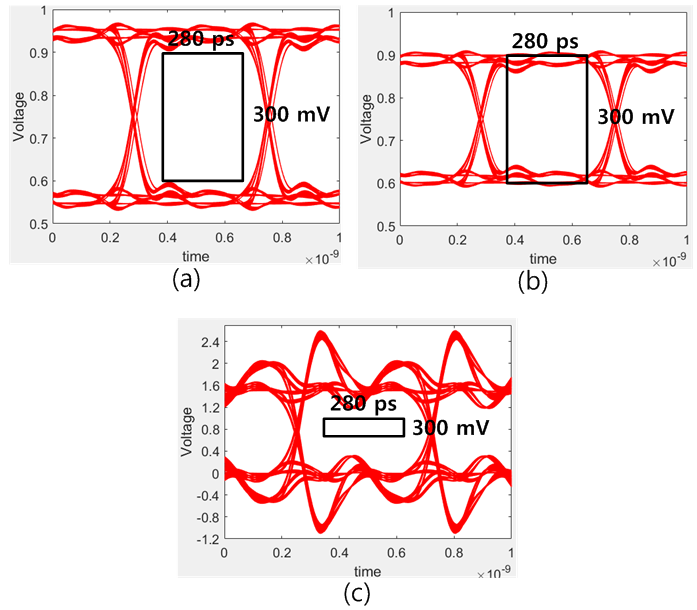##### Fig. 10. RX voltage swing of the DRAM interface with the length of transmission line for 2.133 Gbps PRBS-15 input: (a) write mode for 3 R$_{\mathrm{TX}}$ of the controller, DRAM R$_{\mathrm{RX}}$=60 ${\Omega}$; (b) read mode for 3 R$_{\mathrm{RX}}$ of the controller, DRAM R$_{\mathrm{TX}}$=34 ${\Omega}$.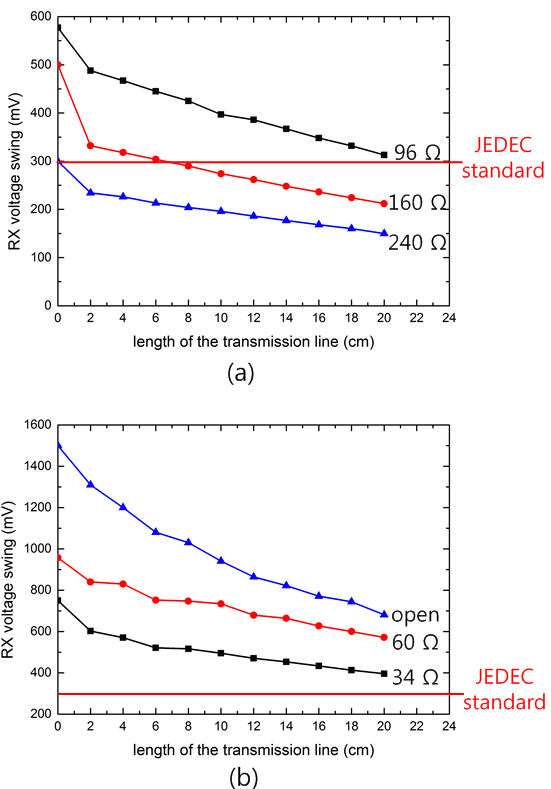## III. MEASUREMENT RESULTS

To verify the power reduction of the DRAM interface by increasing termination resistance, a DRAM controller chip was implemented in a CMOS 65 nm process with an active chip area of 1.64 mm$^{2}$ (LINK: 0.65 mm$^{2}$, serializers and deserializers: 0.08 mm$^{2}$, I/O circuits: 0.65~mm$^{2}$, PLL: 0.09 mm$^{2}$, test module: 0.17 mm$^{2}$, Fig. 11). The supply voltage is 1.5V for the I/O circuits and 1.0V for all other circuits. A commercial 8Gb DDR3 DRAM chip with 16 DQ  was connected to the fabricated DRAM controller chip using a point-to-point interface scheme. To reduce the time skew, all the microstrip lines used for the 16 DQ and 4 DQS lines are designed to have the same length of 25 mm with a standard deviation of 0.013 mm. Also, all the ADDR/CMD lines are 46 mm long with a standard deviation of 0.076 mm (Fig. 12).

A test module is added in the ASIC chip to verify the correct operation of the DRAM controller core block (Fig. 13). The test module generates 128-bit data (eight samples of 16-bit sawtooth wave) at every two periods of the system clock frequency; the system clock is 4 times slower than the data rate of DQ/DQS. The test module sends the 128-bit data to the DRAM controller core along with a 29-bit address and a 1-bit write command every two periods of the system clock. To process the 128-bit data, the DRAM controller core sends 16-bit data eight times through the 16 DQ channels and 4 DQS channels with a burst length of 8; the controller sends one set of 28-bit addresses and commands during the eight 16-bit DQ transactions. By repeating this procedure, the sawtooth data is written to all the 8 Gb of the DRAM chip. After that, the 8 Gb data is read back in a 128-bit unit with a burst length of 8, serialized, and sent to an external logic analyzer to check whether the retrieved data matches the correct sawtooth data. The system clock frequency ranges from 120 MHz to 200 MHz, which corresponds to the data rate in the range from 480 Mbps to 800 Mbps. No error was observed for the R$_{\mathrm{TX}}$ of the DRAM controller up to 160 ${\Omega}$ (bit error rate {\textless} 1.25e-10) during the write mode (Fig. 14) and for the R$_{\mathrm{RX}}$ of the DRAM controller up to infinity (open) during the read mode. Since the larger termination resistance yields the lower power consumption of I/O circuits, the termination resistances (R$_{\mathrm{TX}}$=160 ${\Omega}$, R$_{\mathrm{RX}}$=infinity) are set to the proposed termination resistances in the following measurement of this work.

The power measurement of the DRAM interface revealed that the controller termination resistance of this work (R$_{\mathrm{TX}}$=160 ${\Omega}$, R$_{\mathrm{RX}}$=infinity) yields a 36% reduction in power (Table 3) compared to the default setting of the DRAM controller (R$_{\mathrm{TX}}$=60 ${\Omega}$, R$_{\mathrm{RX}}$=34 ${\Omega}$); R$_{\mathrm{TX}}$=34 ${\Omega}$, R$_{\mathrm{RX}}$=60 ${\Omega}$ for DRAM chip throughout the measurement. The power in Table 3 is measured with an equal number of reads and writes using the maximum bus utilization (94%).

Fig. 15 shows the measured and calculated current of a DQ circuit versus the inverse of the controller termination resistance; Eqs. (17) and (18) are used for the calculation. The range of R$_{\mathrm{TX}}$ and R$_{\mathrm{RX}}$ is chosen to guarantee no error. The measurement and calculation are similar except for the high resistances in read mode (Fig. 15(b)). It is estimated that this discrepancy is caused by the non-linearity of the MOSFET working as the termination resistances and the static current of peripheral circuits.

Table 4 shows the comparison of energy efficiency; the total power of the DRAM interface with an equal number of reads and writes is divided by the multiplication of bandwidth, bus utilization, and DQ width to get the energy efficiency. The energy efficiency of this work (31.3 pJ/b) is less than  and  by 23% and 63%, respectively;  and  reduce power by increasing the refresh period and by voltage scaling, respectively.

##### Table 3. Power budget of DRAM and controller with default and proposed termination resistance of the controller
 Default termination Proposed termination DQ/DQS circuits (DRAM) 175 mW (29.5%) 103 mW (35.1%) DQ/DQS circuits (controller) 221 mW (37.3%) 50 mW (12.9%) ADDR/CMD drivers & logic (DRAM) 90 mW (15.2%) 90 mW (23.8%) ADDR/CMD drivers & logic (controller) 107 mW (18.0%) 107 mW (28.2%) Total power 593 mW 379 mW
##### Table 4. Comparison of energy efficiency with default and proposed termination for controller
 This work   Default termination Proposed termination Bandwidth per DQ 800 Mbps 800 Mbps 1000 Mbps 1333 Mbps DQ width 16 16 8 64 Bus utilization 0.94 0.94 0.6 - Energy efficiency per DQ 48.9 pJ/b 31.3 pJ/b 40.8 pJ/b 83.6 pJ/b
##### Fig. 11. DRAM controller ASIC chip of this work: (a) layout; (b) die photograph.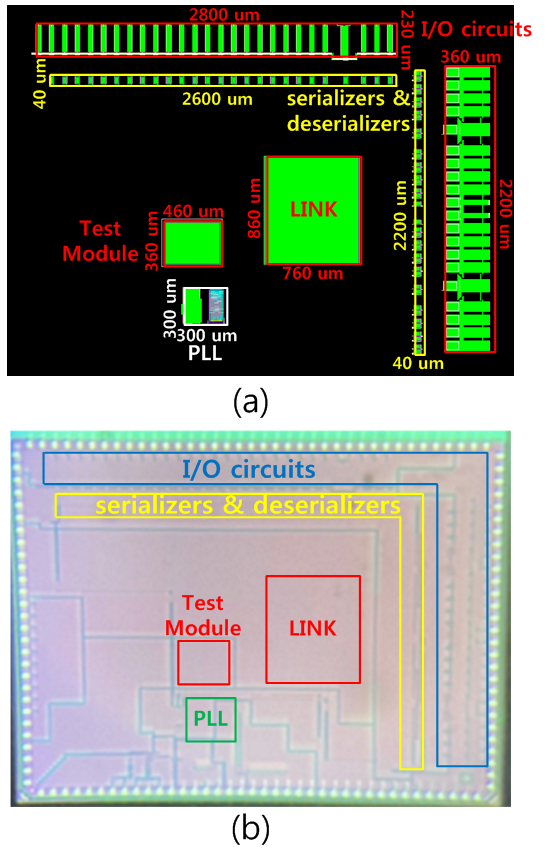##### Fig. 12. Test setup: (a) photograph; (b) PCB layout.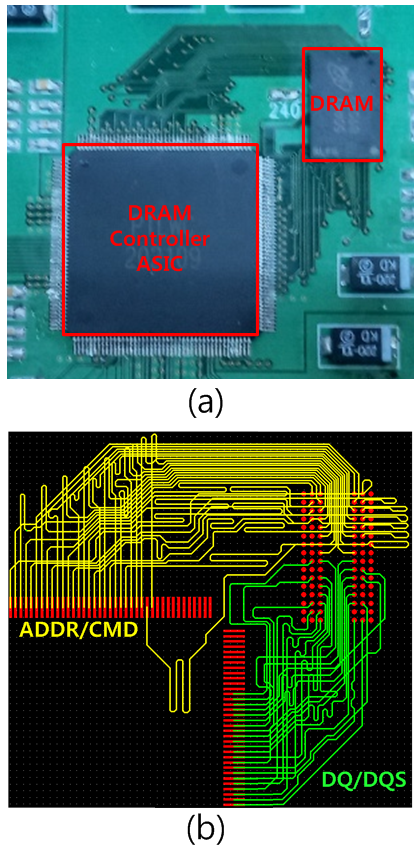##### Fig. 14. Bit Error Rate versus R$_{\mathrm{TX}}$ of the DRAM controller for 800 Mbps saw-tooth wave.##### Fig. 15. Comparison of measured (solid line) and calculated (dashed line) current of a DQ circuit versus termination resistances of the controller with channel length = 25 mm (t$_{\mathrm{f}}$/t$_{\mathrm{ui}}$=0.12): (a) write mode; (b) read mode.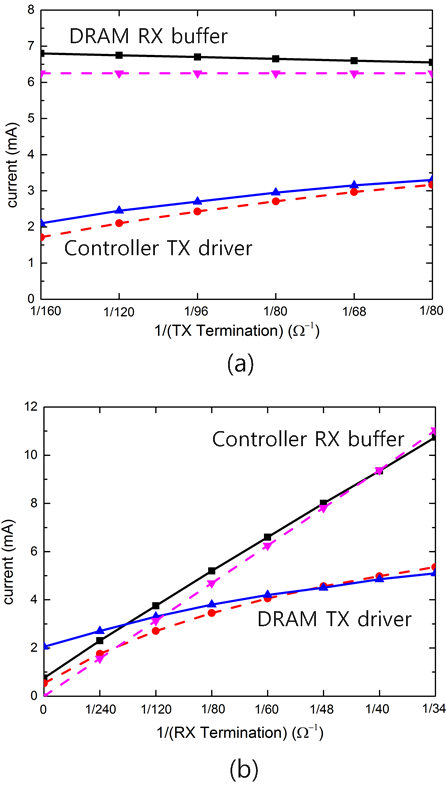## IV. APPLICATION TO LONG-REACH POINT-TO-POINT AND MULTI-DROP DRAM INTERFACE

The original target of this work is a short-reach low-power point-to-point DRAM interface on a motherboard PCB by increasing the termination resistance of the DRAM controller. To probe the potential application of this work to long-reach point-to-point and multi-drop DRAM interface, S-parameters were measured on FR4 microstrip lines with the length ranging from 10cm to 100cm, and the parameters of the lossy transmission line model (HSPICE W model) were extracted from the measured S-parameters, as follows (L$_{0}$=316 nH/m, C$_{0}$=123 pF/m, R$_{0}$=0.598 ${\Omega}$/m, G$_{0}$=0 nS/m, R$_{\mathrm{s}}$=1.52 m${\Omega}$/m/${\sqrt{}}$Hz, G$_{\mathrm{d}}$=14.2 pS/m/Hz). Comparison of the measured eye diagrams with the HSPICE simulation using the extracted parameters confirmed the accuracy of the extracted model parameters (Fig. 16).

##### Fig. 16. Comparison of eye diagrams between HSPICE simulation and measurement at receiver input with FR4 microstrip lines and R$_{\mathrm{TX}}$=R$_{\mathrm{RX}}$=50 ${\Omega}$.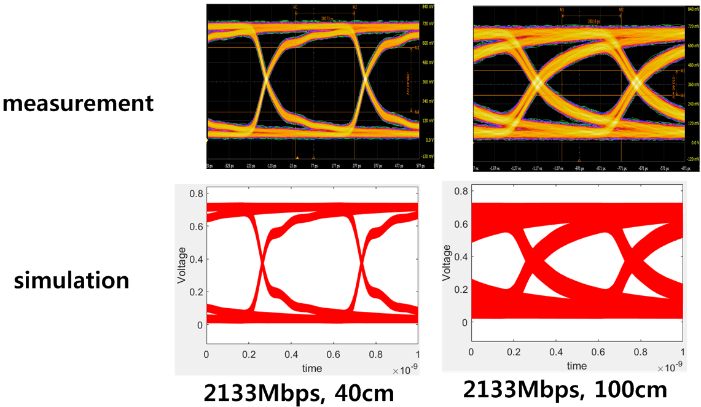### A. Long-reach Point-to-point DRAM Interface

With the short-reach interconnect, the transmission line can be considered a lumped circuit and reflection does not matter. With the very long interconnect, loss dominates reflection. In the intermediate-length interconnect, reflection dominates loss, as shown in Fig. 17 (20 cm long interconnect), where large reflection occurs at the receiver input for the read mode. The maximum data rate versus the interconnect length was presented in Fig. 18, where the eye mask of the JEDEC standard at receiver input was used as the criterion for successful data transmission.

##### Fig. 17. Eye diagrams (simulation) at receiver input with 20 cm microstrip line at 800 Mbps PRBS-15 data: (a) write mode with R$_{\mathrm{TX\_CONT}}$=160${\Omega}$, R$_{\mathrm{RX \_ DRAM}}$=60${\Omega}$; (b) read mode with R$_{\mathrm{RX \_ CONT}}$=infinity, R$_{\mathrm{TX \_ DRAM}}$=34${\Omega}$.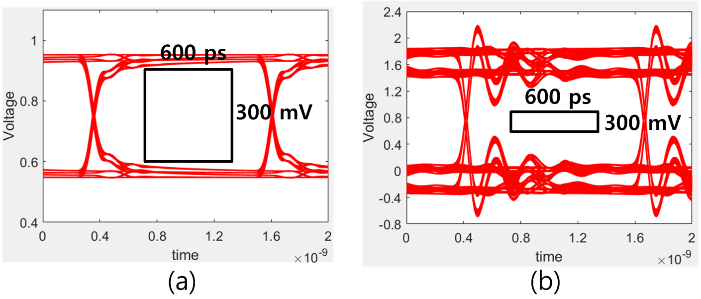##### Fig. 18. Maximum data rate versus channel length of the point-to-point interface: (a) write mode, R$_{\mathrm{TX}}$=160 ${\Omega}$, 120 ${\Omega}$, 34 ${\Omega}$, R$_{\mathrm{RX}}$=60 ${\Omega}$; (b) read mode, R$_{\mathrm{TX}}$=34 ${\Omega}$, R$_{\mathrm{RX}}$=infinity, 60 ${\Omega}$.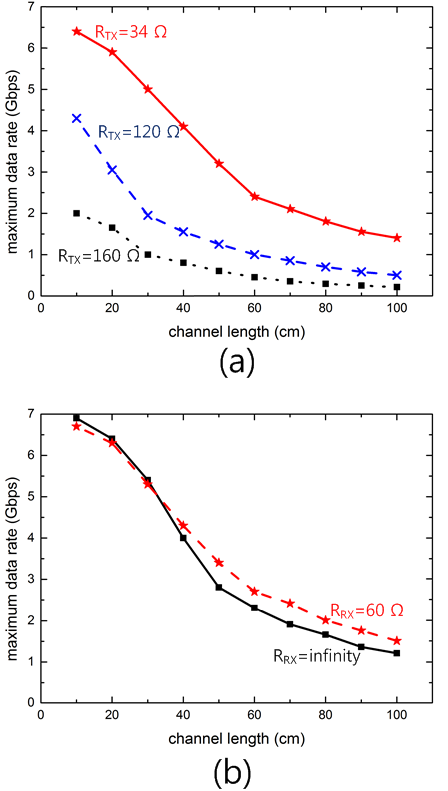### B. Multi-drop DRAM Interface

The approach of this work to reduce power consumption by increasing the termination resistance of the DRAM controller was applied to a multi-drop DRAM interface (Fig. 19); a stub-series terminated logic (SSTL) was used to reduce reflection . The maximum data rate versus the termination resistance of the DRAM controller (Fig. 22) was obtained by applying the eye mask of the JEDEC standard to the simulated eye diagrams for the write mode (Fig. 20) and read mode (Fig. 21), respectively. The read mode works over 2 Gbps with 2 DIMMs and R$_{\mathrm{RX \_ CONT}}$=infinity (Fig. 22(b)). However, for the successful write mode, R$_{\mathrm{TX \_ CONT}}$ should be reduced significantly from 160 ${\Omega}$, the proposed value in this work (Fig. 22(a)).

##### Fig. 19. Multi-drop DRAM interface, two dual-rank DIMMs, SSTL.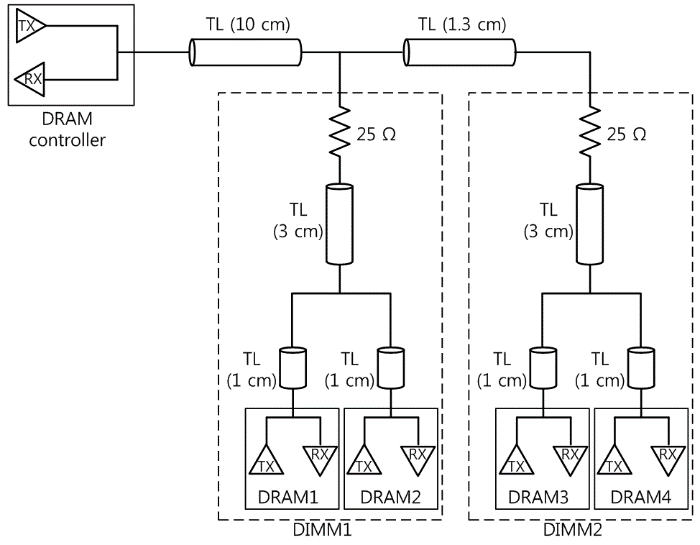##### Fig. 20. Write mode with 2 DIMMs, eye diagram (simulation) at RX input, R$_{\mathrm{TX \_ CONT}}$=48 ${\Omega}$, R$_{\mathrm{RX \_ DRAM}}$=60 ${\Omega}$ (target DRAM), 120 ${\Omega}$ (other DRAMs), at 2.133 Gbps PRBS-15: (a) write to DIMM1; (b) write to DIMM2.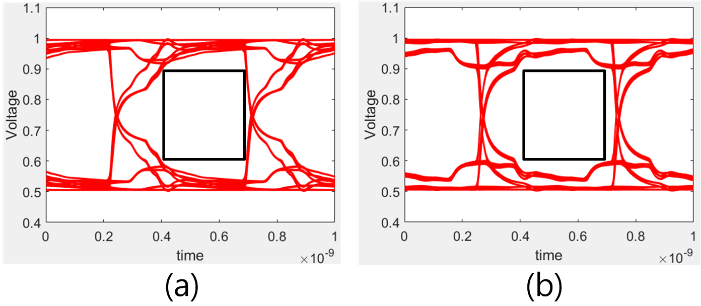##### Fig. 21. Read mode with 2 DIMMs, eye diagram (simulation) at RX input, R$_{\mathrm{RX \_ CONT}}$=infinity, R$_{\mathrm{TX \_ DRAM}}$ =34 ${\Omega}$ (target DRAM), 120 ${\Omega}$ (other DRAMs), at 2.133 Gbps PRBS-15: (a) read from DIMM1; (b) read from DIMM2.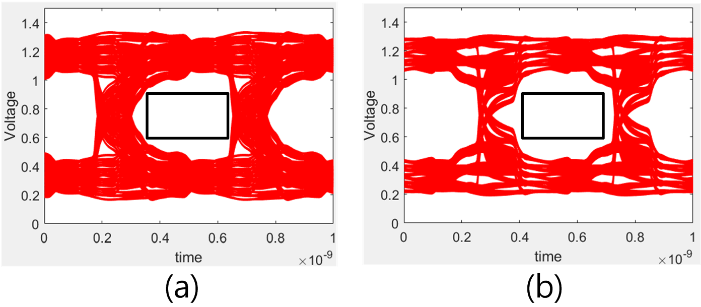##### Fig. 22. Maximum data rate of multi-drop DRAM interface versus the termination resistance of the DRAM controller, dual-rank DIMM: (a) write mode; (b) read mode.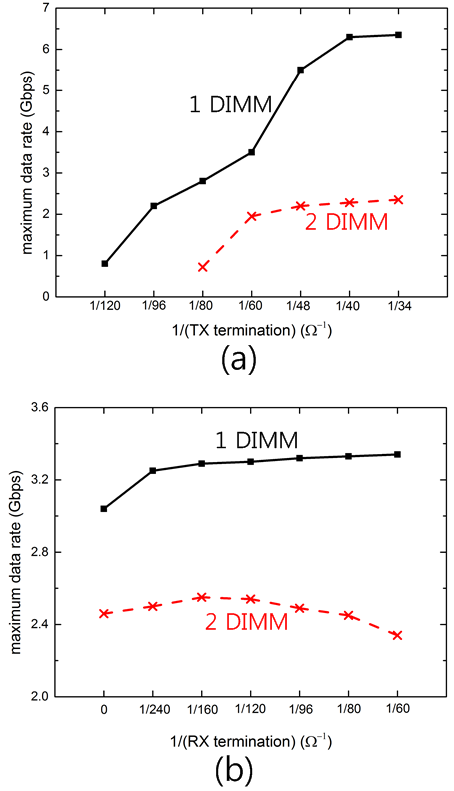## V. CONCLUSIONS

A low-power DRAM controller ASIC is proposed for on-device deep learning applications with short-reach interconnects, where the I/O power takes a significant portion of the entire system power. To reduce the I/O power, the termination resistance of the DRAM controller is increased up to the point where the RX signal swing reaches the minimum swing for correct data recovery; the minimum RX signal swing is defined by the JEDEC standard. A DRAM controller ASIC chip was implemented in a 65 nm CMOS process to verify the low-power DRAM I/O interface. A commercial 8Gb DDR3 DRM chip was connected to the controller ASIC chip for measurement. The TX and RX termination resistance was set to 160 ${\Omega}$ and infinity for the DRAM controller and they are set to the default values of the JEDEC standard, 34 ${\Omega}$ and 60 ${\Omega}$ for the DDR3 DRAM chip. A point-to-point short reach interconnect of 25 mm DQ/DQS line is used with FR-4 PCB channel to avoid the signal integrity issues; the interconnect length can be extended up to 6 cm at the data rate of 2.133 Gbps according to simulation with the proposed termination of the controller (R$_{\mathrm{TX}}$=160 ${\Omega}$, R$_{\mathrm{RX}}$=infinity). The proposed DRAM controller ASIC chip occupies an active area of 1.64 mm$^{2}$ in a 65 nm process with 16 DQ 8 Gb configuration; it works at the data rate of 800 Mbps per DQ pin. Using the proposed controller termination, the DRAM interface consumes 379 mW with an equal number of reads and writes; the active power is reduced by 36% compared to the default termination of the JEDEC standard (R$_{\mathrm{TX}}$=60 ${\Omega}$, R$_{\mathrm{RX}}$=34 ${\Omega}$ for the controller). Equations are derived for the TX and RX current of the DRAM interface with a point-to-point CTT interconnect. For the clock signal such as DQS, the derived equation reveals that the TX current is minimized when the time of flight of the PCB channel is integer multiples of the half period of the clock signal with the large TX and RX terminations (R$_{\mathrm{TX}}$${\geq}50 {\Omega}, R_{\mathrm{RX}}$${\geq}$50 ${\Omega}$); this is due to the recycling of the channel charge.

## ACKNOWLEDGMENTS

This work was supported by National Research Foundation (NRF) grant funded by the Korea government (NRF-2019R1A5A1027055), the National Research Foundation of Korea (NRF) grant funded by the Korea government (MSIT) (NRF-2022R1A2C2003451) and National R&D Program through the National Research Foundation of Korea (NRF) funded by Ministry of Science and ICT (2020M3H2A107804514).

## References

1
A. E. Eshratifar, A. Esmaili, and M. Pedram, “BottleNet: A deep learning architecture for intelligent mobile cloud computing services,” IEEE/ACM International Symposium on Low Power Electronics and Design (ISLPED), pp. 1-6, Jul. 2019.2
W.-J. Chang et al., “iBuffet: A deep learning-based intelligent calories management system for eating buffet meals,” IEEE International Conference on Consumer Electronics (ICCE), pp. 1-2, Jan. 2021.3
B. Fang et al., “FlexDNN: Input-Adaptive On-Device Deep Learning for Efficient Mobile Vision,” IEEE/ACM Symposium on Edge Computing (SEC), pp. 84-95, Feb. 2021.4
J. Lee and H. -J. Yoo, "An Overview of Energy-Efficient Hardware Accelerators for On-Device Deep-Neural-Network Training," IEEE Open Journal of the Solid-State Circuits Society, vol. 1, pp. 115-128, Oct. 2021.5
N. P. Jouppi et al., "In-datacenter performance analysis of a tensor processing unit," ACM/IEEE 44th Annual International Symposium on Computer Architecture (ISCA), pp. 1-12, Dec. 2017.6
J. Wang, S. Park and C. S. Park, "Optimization of Communication Schemes for DMA-Controlled Accelerators," in IEEE Access, vol. 9, pp. 139228-139247, Oct. 2021.7
DDR3 SDRAM JEDEC standard, JESD79-3C, Nov. 2008.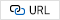8
S. M. JAFRI et al., “Refresh Triggered Computation: Improving the Energy Efficiency of Convolutional Neural Network Accelerators,” ACM Transactions on Architecture and Code Optimization (TACO), Vol. 18, pp. 1-29, Dec. 2020.9
F. Schuiki, M. Schaffner, F. K. Gürkaynak and L. Benini, "A Scalable Near-Memory Architecture for Training Deep Neural Networks on Large In-Memory Datasets," IEEE Transactions on Computers, vol. 68, pp. 484-497, April. 2019.10
C. Sudarshan et al., “A Lean, Low Power, Low Latency DRAM Memory Controller for Transprecision Computing,” Embedded Computer Systems: Architectures, Modeling, and Simulation. (SAMOS), Aug. 2019.11
E. Mintarno and S. Y. Ji, "Bit-pattern sensitivity analysis and optimal on-die-termination for high-speed memory bus design," IEEE 18th Conference on Electrical Performance of Electronic Packaging and Systems, pp. 199-202, Nov. 2009.12
Performance Characteristics of IC Packages, 2000 Packaging Data book, Intel.13
G. Dong, Y. Biao, D. Xidong and L. Yuan,, "Research on the influence of vias on signal transmission in multi-layer PCB," 13th IEEE International Conference on Electronic Measurement & Instruments (ICEMI), pp. 406-409, Oct. 2017.15
DDR3L SDRAM description, MT41K512M16, Micron, 2015.16
S. Y. Ji, B. Loop, P. D. James and V. Paranjape, "An empirical study of performance and power scaling of low voltage DDR3," 19th Topical Meeting on Electrical Performance of Electronic Packaging and Systems, pp. 9-12, Nov. 2010.17
W. -C. Lee et al., "Parallel Branching of Two 2-DIMM Sections With Write-Direction Impedance Matching for an 8-Drop 6.4-Gb/s SDRAM Interface," in IEEE Transactions on Components, Packaging and Manufacturing Technology, vol. 9, no. 2, pp. 336-342, Feb. 2019.Won-Cheol Lee received the B.S. degree in Electronic and Electrical Engineering from Pohang University of Science and Technology (POSTEH), Pohang, Korea, in 2015. Currently, he is pursuing the M.S and Ph.D. degree at POSTECH. His research interests include DRAM controller and hardware accelerators.

Ho-Jun Kim received the B.S. degree in Electronic and Electrical Engineering from Hongik University, Seoul, Korea, in 2019 and the M.S. degree in Electronic and Electrical Engineering from the Pohang University of Science and Technology (POSTEH), Pohang, Korea, in 2021. Currently, he is pursuing the Ph.D. degree at POSTECH. His research interests include DRAM controller.

Hong-June Park (Senior Member, IEEE) received the B.S. degree from the Department of Electronic Engi-neering, Seoul National University, Seoul, Korea, the M.S. degree from the Korea Advanced Institute of Science and Technology (KAIST), Taejon, Korea, and the Ph.D. degree from the Department of Electrical Engineering and Computer Sciences, University of California, Berkeley, Berkeley, CA, USA, in 1979, 1981, and 1989, respectively. He was a CAD Engineer with ETRI, Korea, from 1981 to 1984 and a Senior Engineer in the TCAD Department of Intel Corporation from 1989 to 1991. In 1991, he joined the Faculty of Electronic and Electrical Engineering, Pohang University of Science and Technology (POSTECH), Pohang, Korea, where he is currently a Professor. His research interests include CMOS analog circuit design such as high-speed interface circuits, ROIC of touch sensors, and analog/digital beamformer circuits for ultrasound medical imaging. Dr. Park is a member of IEEK. He served as the Editor-in-Chief of the Journal of Semiconductor Technology and Science, an SCIE journal (http://www.jsts.org), from 2009 to 2012, as the Vice President of IEEK in 2012, and as a technical program committee member of ISSCC, SOVC, and A-SSCC for several years.